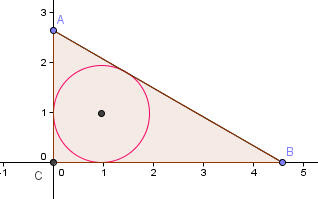## Saturday 19 September 2009

### A Trio of Triangle ProblemsIn the January, 1929, issue of the American Mathematical Monthly there appears a problem submitted by J. Rosenbaum of Milford Connecticut. The problem begins, "It is well known that the radius of the inscribed circle of a right triangle is equal to half the difference between the sums of the legs and the hypotenuse." I ignore for now the problem posted, but suggest that the theorem suggested may be less well known now than it might have been in the past. But the challenge... prove it is true. I won't spoil it now, and will wait for a reader to supply a good proof... and if none appears, I will post a proof here in a day or two.A second "well known" theorem in the same article included a theorem I call a "Pythagorean look-alike". In a right tetrahedron [a triangular pyramid in which three right angles meet at a single vertex... imagine one point, A, being at the origin of the three-dimensional coordinate grid and points A, B, and C being on the x, y, and z axes at (b,0,0,) (0,c,0) and (0,0,d) ] Then the sum of the squares of the areas of the three triangles meeting at the triple right vertex is equal to the square of the area of the triangle BCD. Once more the challenge is to derive the given relationship.A popular theorem to introduce to students is Napoleon's Theorem: if equilateral triangles are constructed on the three legs of any triangle, the centers of the three new triangles will also form an equilateral triangle. You can actually do that two ways, by constructing them on the exterior of the triangle (shown above), or by constructing them on the interior sides of each leg. So here is the problem, what is the difference in the areas of the equilateral triangles on the exterior and interior.